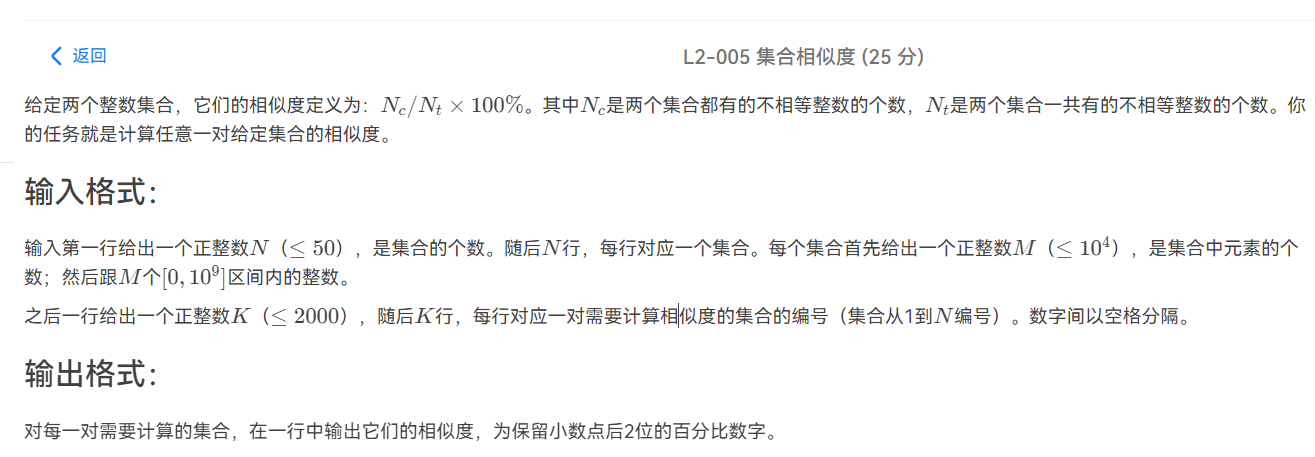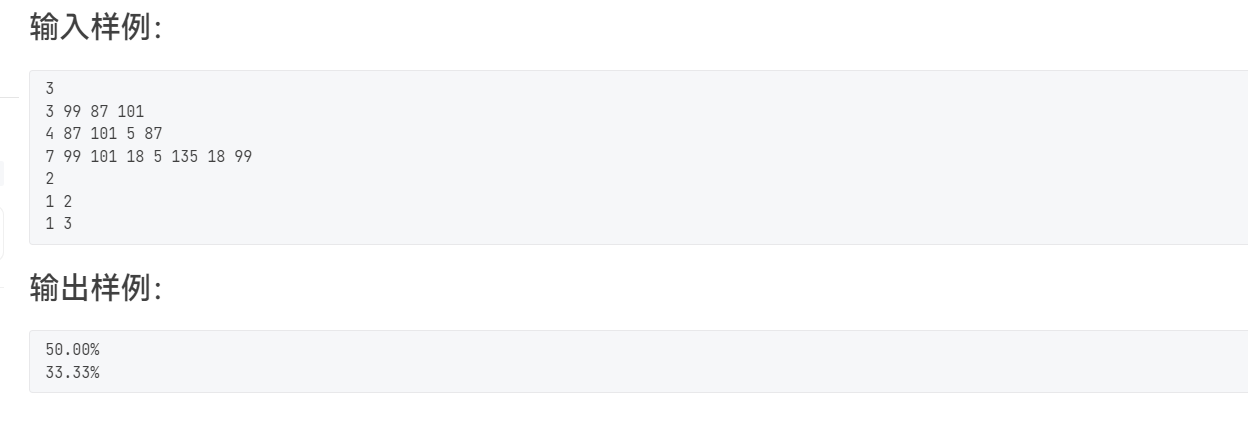• 2020-04-27 22:26:37

给定两个整数集合，它们的相似度定义为：N​c​​/N​t​​×100%。其中N​c​​是两个集合都有的不相等整数的个数，N​t​​是两个集合一共有的不相等整数的个数。你的任务就是计算任意一对给定集合的相似度。

### 输入格式：

输入第一行给出一个正整数N（≤50），是集合的个数。随后N行，每行对应一个集合。每个集合首先给出一个正整数M（≤10​4​​），是集合中元素的个数；然后跟M个[0,10​9​​]区间内的整数。

之后一行给出一个正整数K（≤2000），随后K行，每行对应一对需要计算相似度的集合的编号（集合从1到N编号）。数字间以空格分隔。

### 输出格式：

对每一对需要计算的集合，在一行中输出它们的相似度，为保留小数点后2位的百分比数字。

### 输入样例：

3
3 99 87 101
4 87 101 5 87
7 99 101 18 5 135 18 99
2
1 2
1 3


### 输出样例：

50.00%
33.33%

50.00%
33.33%

//tle的代码，主要是为了熟悉数组去重的使用
#include<iostream>
#include<algorithm>
#include<cstring>
#include<algorithm>
#include<cstdio>
#include<vector>

using namespace std;

vector<int>vec;
int n;

int main()
{
cin>>n;
for(int i=1; i<=n; i++)
{
int sizes=0;
cin>>sizes;
for(int j=0; j<sizes; j++)
{
int tmp;
cin>>tmp;
vec[i].push_back(tmp);
}
//数组去重

sort(vec[i].begin(),vec[i].end());
vec[i].erase(unique(vec[i].begin(),vec[i].end()),vec[i].end());
//cout<<vec[i].size()<<endl;
}

cin>>n;

while(n--)
{
int nc=0,nt=0;
int a,b;
cin>>a>>b;
for(int i=0 ;i<vec[a].size();i++)
{
for(int j=0 ; j<vec[b].size(); j++)
{
if(vec[a][i]==vec[b][j])
{
nc++;
break;
}
}

}
nt=vec[a].size()+vec[b].size()-nc;
//cout<<nc<<" "<<nt<<endl;
double ans=nc*1.0/nt;
ans*=100;
printf("%.2f",ans);
printf("%\n");

}

}
//ac代码，利用set集合
#include<iostream>
#include<algorithm>
#include<cstdio>
#include<cstring>
#include<set>

using namespace std;

set<int>s;
int n;

int main()
{

cin>>n;
for(int i=1; i<=n; i++)
{
int sizes;
cin>>sizes;
for(int j=0; j<sizes; j++)
{
int tmp;
cin>>tmp;
s[i].insert(tmp);
}
}
cin>>n;
while(n--)
{
int a,b;
cin>>a>>b;
int nc=0,nt=0;
for(set<int>::iterator it1=s[a].begin();it1!=s[a].end();it1++)
{
int tmp=*it1;
if(s[b].find(tmp)!=s[b].end())
{
nc++;
}

}
nt=s[a].size()+s[b].size()-nc;
//cout<<nc<<" "<<nt<<endl;
double ans=nc*1.0/nt;
ans*=100;
printf("%.2f",ans);
printf("%\n");
}

}



更多相关内容
• L2-005 集合相似度 时间限制 400 ms 内存限制 64 MB 题目描述： 给定两个整数集合，它们的相似度定义为：Nc/Nt∗100%N_{c} / N_{t} * 100\%Nc​/Nt​∗100%。其中N c 是两个集合都有的不相等整数的个数，NtN_{t}Nt...

L2-005 集合相似度

时间限制
400 ms
内存限制
64 MB

题目描述：

给定两个整数集合，它们的相似度定义为： N c / N t ∗ 100 % N_{c} / N_{t} * 100\% 。其中N c 是两个集合都有的不相等整数的个数， N t N_{t} 是两个集合一共有的不相等整数的个数。你的任务就是计算任意一对给定集合的相似度。

输入格式：
输入第一行给出一个正整数N（≤50），是集合的个数。随后N行，每行对应一个集合。每个集合首先给出一个正整数M（≤ 1 0 4 10^{4} ），是集合中元素的个数；然后跟M个[0, 1 0 9 10^{9} ]区间内的整数。

之后一行给出一个正整数K（≤2000），随后K行，每行对应一对需要计算相似度的集合的编号（集合从1到N编号）。数字间以空格分隔。

输出格式：
对每一对需要计算的集合，在一行中输出它们的相似度，为保留小数点后2位的百分比数字。

输入样例：
3
3 99 87 101
4 87 101 5 87
7 99 101 18 5 135 18 99
2
1 2
1 3
输出样例：
50.00%
33.33%

给定n个集合
再给定k组 要进行计算相似度的两个集合
求出 这 两 个 集 合 不 重 复 的 相 同 的 个 数 这 两 个 集 合 中 不 重 所 有 个 数 \frac{这两个集合不重复的相同的个数}{这两个集合中不重所有个数}

emmmmmmm

set将每个集合弄成不重复的
然后 一个一个去填充到res中

两个集合相同的个数 = 两个集合的个数 - 两个集合合并之后不同的个数
答案就是 两 个 集 合 相 同 的 数 两 个 集 合 合 并 之 后 不 同 的 个 数 \frac{两个集合相同的数}{两个集合合并之后不同的个数}

注: java 会t 数据量有点大

暴力

import java.io.*;
import java.math.*;
import java.util.*;

public class Main
{

public static void main(String[] args)
{
int n = sc.nextInt();
TreeSet<Integer> shu[] = new TreeSet[n + 10];

for (int i = 1; i <= n; i++)
{
int cnt = sc.nextInt();
shu[i] = new TreeSet<Integer>();
while (cnt-- > 0)
{
int x = sc.nextInt();
}
}

int k = sc.nextInt();
TreeSet<Integer> res = new TreeSet<Integer>();
while (k-- > 0)
{
int a = sc.nextInt();
int b = sc.nextInt();

res.clear();
for (int i : shu[a])
for (int i : shu[b])

out.printf("%.2f%%\n", (shu[a].size() + shu[b].size() - res.size()) * 100.0 / res.size());
}

out.flush();
out.close();
}

static Scanner sc = new Scanner(System.in);
static PrintWriter out = new PrintWriter(System.out);
}



import java.io.*;
import java.math.*;
import java.util.*;

public class Main
{

public static void main(String[] args)
{
int n = sc.nextInt();
TreeSet<Integer> shu[] = new TreeSet[n + 10];

for (int i = 1; i <= n; i++)
{
int cnt = sc.nextInt();
shu[i] = new TreeSet<Integer>();
while (cnt-- > 0)
{
int x = sc.nextInt();
}
}

int k = sc.nextInt();
TreeSet<Integer> res = new TreeSet<Integer>();
while (k-- > 0)
{
int a = sc.nextInt();
int b = sc.nextInt();

res.clear();

out.printf("%.2f%%\n", (shu[a].size() + shu[b].size() - res.size()) * 100.0 / res.size());
}

out.flush();
out.close();
}

static Scanner sc = new Scanner(System.in);
static PrintWriter out = new PrintWriter(System.out);
}



TreeSet

如果有说错的 或者 不懂的 尽管提 嘻嘻

一起进步！！！

闪现

展开全文学习
• pta集合相似度 给定两个整数集合，它们的相似度定义为：Nc​​ /N​t×100%。其中N​c是两个集合都有的不相等整数的个数，Nt是两个集合一共有的不相等整数的个数。你的任务就是计算任意一对给定集合的相似度。 输入...

## pta集合相似度

给定两个整数集合，它们的相似度定义为：Nc​​ /N​t×100%。其中N​c是两个集合都有的不相等整数的个数，Nt是两个集合一共有的不相等整数的个数。你的任务就是计算任意一对给定集合的相似度。

输入格式：
输入第一行给出一个正整数N（≤50），是集合的个数。随后N行，每行对应一个集合。每个集合首先给出一个正整数M（≤10000），是集合中元素的个数；然后跟M个[0,10^9 ]区间内的整数。
之后一行给出一个正整数K（≤2000），随后K行，每行对应一对需要计算相似度的集合的编号（集合从1到N编号）。数字间以空格分隔。

输出格式：
对每一对需要计算的集合，在一行中输出它们的相似度，为保留小数点后2位的百分比数字。

输入样例：
3
3 99 87 101
4 87 101 5 87
7 99 101 18 5 135 18 99
2
1 2
1 3
输出样例：
50.00%
33.33%

一开始打算用c写结果弄了半天，最后一个点仍然超时……
感觉是查找相同数字的嵌套循环太耗时间了。

#include <stdio.h>
#include <stdlib.h>

float same(int a[],int b[], int lena, int lenb);//找出相同的数返回相同数的个数

int main(void){
int n,m,i,j,k,temp;
float x,y;
int mark = 1;
int **space,*len,**request;//space储存集合，len存储space的长度，request存储要查询的集合名

scanf("%d",&n);//输入集合个数
len = (int*)malloc(n*sizeof(int));
space = (int **)malloc(n*sizeof(int *));
for (i = 0;i < n; i++){
scanf("%d",&len[i]);
space[i] = (int *)malloc(len[i]*sizeof(int ));
for(j = 0; j < len[i];j ++){
scanf("%d",&temp);//输入将要放入集合的数
if(j > 0){
for(k = 0; k <= j-1; k ++){
if (space[i][k] == temp){
len[i]--;
mark = 0;
}
}
}
if (mark == 0){
mark = 1;
j--;
space[i] = (int *)realloc(space[i], len[i]*sizeof(int ));
} else{
space[i][j] = temp;
}
}
space[i] = (int *)realloc(space[i],len[i]*sizeof(int ));
}
scanf("%d",&m);
request = (int**) malloc(m*sizeof(int*));
for(i = 0; i < m; i++){
request[i] = (int*)malloc(2*sizeof(int));
scanf("%d %d",&request[i],&request[i]);
}
for(i = 0; i < m; i++){
x = same(space[request[i]-1],space[request[i]-1],len[request[i]-1],len[request[i]-1]);
y = len[request[i]-1]+len[request[i]-1]-x;
printf("%.2f%%\n",(x/y)*100);
}
return 0;
}

float same(int a[],int  b[], int lena, int lenb){
int i,j;
float z = 0;
for(i = 0; i < lena; i++){
for (j = 0; j < lenb; j++) {
if (a[i] == b[j]){
z ++;
}
}
}
return z;
}



后来放弃了用c……改用Python来写结果不到20行完美通过…………

N = input()  # 输入集合个数
lists = []  # 集合列表
for i in range(int(N)):
temper = input()  # 输入n个集合
lists.append(set(list(temper.split())[1:])) # 将n个集合加入集合列表

M = input()
list1 = []  # 要计算的集合组
for i in range(int(M)):
temper = input()
list1.append(temper.split())

for i in range(int(M)):
k = len(lists[int(list1[i])-1] & lists[int(list1[i])-1])
z = len(lists[int(list1[i])-1] | lists[int(list1[i])-1])
print(str(format(float(k)/float(z)*100,'.2f'))+"%")



最后一句人生苦短我用Python！

展开全文python 算法
• 分析 java超时 import java.util.ArrayList; import java.util.HashSet; import java.util.Scanner; public class Main { ... public static void main(String[] args) { ... //一维放的集合，二维放的集.## 分析

### java超时

1. 用ArrayList去嵌套一个HashSet集合，去存放每个集合，以及他们的元素，因为输入时可能也会出现重复元素，于是用set；
2. 然后在处理的时候，我用一个HashSet集合all去存放两个集合的所有元素，用一个HashSet集合temp去存放第一个集合的元素；
3. 我通过两次循环，第一次把第一个集合的所有元素加进all和temp中；第二次也把所有元素加进了all中，然后向temp中添加第二个集合的元素，如果添加失败，那么就是共同元素，用一个变量common去记录；
4. 最后输出的时候，我们需要用两个%%来表示百分号；

import java.util.ArrayList;
import java.util.HashSet;
import java.util.Scanner;

public class Main {

public static void main(String[] args) {
Scanner sc = new Scanner(System.in);
int n = sc.nextInt();
//一维放的集合，二维放的集合里面元素
ArrayList<HashSet<Integer>> list = new ArrayList<>();
for (int i = 0; i < n; i++) {
int m = sc.nextInt();
for (int j = 0; j < m; j++) {
}
}
int k = sc.nextInt();
for (int i = 0; i < k; i++) {
//将其中一个集合的所有元素放进一个set，然后把另一个集合的元素也加在set里，统计加不进去的次数，就是两个集合的共有的不同元素
HashSet<Integer> temp = new HashSet<>();
HashSet<Integer> all = new HashSet<>();//两个集合一共有的不相等整数的元素
int common = 0;
int x = sc.nextInt();
int y = sc.nextInt();
for (Object o : list.get(x - 1)) {//遍历第x个集合
}
for (Object o : list.get(y - 1)) {//遍历第y个集合
if (temp.add((Integer) o) == false) {//说明这个元素没加进去，是共有元素
common++;
}
}
System.out.printf("%.2f%%\n", 100.0 * common / all.size());
}
}
}



### c++ AC

1. 刚开始和上面的java同思路，如下面注释的那一段，但是最后一个测试点超时了，可能内部两遍循环，比较费时；
2. 然后参考了网上大佬的思路，就是用一个变量all去记录两个集合的不相等的元素总数，我们可以把v[b-1]，这个集合的大小赋给all，然后遍历v[a-1]这个集合，利用find函数，如果v[a-1]中的元素不在v[b-1]集合中，那么就会返回v[b - 1].end()；
3. 如果v[a-1]中的元素不在v[b-1]集合中，那就all++，否则就是为共同元素，用一个commo变量去记录；
4. 新学到的set知识点：
4.1 temp.insert(o).second == false：表示temp这个set集合，添加元素失败
4.2 v[b - 1].find(o) == v[b - 1].end()，表示v[b-1]这个set集合没有查找到o这个元素；
4.3 vector<set> v(n)，需要指明向量的长度，这个n刚开始没加，就出错了，不太理解，有会的大佬可以告知一下；
#include<bits/stdc++.h>

using namespace std;

int main() {

int n;
cin >> n;
vector<set<int>> v(n);
for (int i = 0; i < n; i++) {
int m;
cin >> m;
for (int j = 0; j < m; j++) {
int val;
cin >> val;
v[i].insert(val);
}
}
int k;
cin >> k;
for (int i = 0; i < k; i++) {
int a, b;
cin >> a >> b;

//同java思路，但最后一个点超时
//		set<int> all;
//		set<int> temp;
//		int common = 0;
//		for (auto o : v[a - 1]) {
//			temp.insert(o);
//			all.insert(o);
//		}
//		for (auto o : v[b - 1]) {
//			if (temp.insert(o).second == false) {//表明添加失败
//				common++;
//			}
//			all.insert(o);
//		}
//		printf("%.2lf%\n", 100.0 * common / all.size());
int common = 0;//共同元素
int all = v[b - 1].size();//两个集合全部的元素 ，放的是b-1的，那么遍历a-1，看看与b-1集合有几个共同的
for (auto o : v[a - 1]) {
if (v[b - 1].find(o) == v[b - 1].end()) { //没有找到共同元素
all++;
} else {
common++;//共同的元素
}
}

printf("%.2lf%\n", 100.0 * common /  all);
}
return 0;
}

展开全文java
• L2-005 集合相似度 （25 分） 给定两个整数集合，它们的相似度定义为：N​c​​/N​t​​×100%。其中N​c​​是两个集合都有的不相等整数的个数，N​t​​是两个集合一共有的不相等整数的个数。你的任务就是计算...c++ 算法
• 之后一行给出一个正整数K（≤2000），随后K行，每行对应一对需要计算相似度集合的编号（集合从1到N编号）。数字间以空格分隔。 输出格式： 对每一对需要计算的集合，在一行中输出它们的相似度，为保留小数点后2...java python
• 给定两个整数集合，它们的相似度定义为：Nc​/Nt​×100%。其中Nc​是两个集合都有的不相等整数的个数，Nt​是两个集合一共有的不相等整数的个数。你的任务就是计算任意一对给定集合相似度。 输入格式： 输入第...
• 这个题目就是求两个集合的并集的不同数字个数和两个集合都有的不同数字个数。 def count(a,b): a1=set(a[1:]) b1=set(b[1:]) cn=0 for i in a1: if i in b1: cn+=1 com=[] com.extend(a[1:]) com.extend(b...python 算法
• 7-8 集合相似度 (25分) 快速跳转 问题 我的问题代码 原因 AC代码 给定两个整数集合，它们的相似度定义为：N ​c ​​ /N ​t ​​ ×100%。其中N ​c ​​ 是两个集合都有的不相等整数的个数，N ​t ​​ 是两个集...c++ set
• 给定两个整数集合，它们的相似度定义为：N​c​​/N​t​​×100%。其中N​c​​是两个集合都有的不相等整数的个数，N​t​​是两个集合一共有的不相等整数的个数。你的任务就是计算任意一对给定集合相似度。 输入...c语言
• L2-005 集合相似度 (25 分) 给定两个整数集合，它们的相似度定义为：Nc/Nt×100%。其中N c是两个集合都有的不相等整数的个数，Nt是两个集合一共有的不相等整数的个数。你的任务就是计算任意一对给定集合的相似度。 ...c++ 算法 c语言
• 给定两个整数集合，它们的相似度定义为：N c ​ /N t ​ ×100%。其中N c ​ 是两个集合都有的不相等整数的个数，N t ​ 是两个集合一共有的不相等整数的个数。你的任务就是计算任意一对给定集合相似度。 输入格式...c语言 算法 动态规划
• ----喻言 给定两个整数集合，它们的相似度定义为：N​c​​/N​t​​×100%。其中N​c​​是两个集合都有的不相等整数的个数，N​t​​是两个集合一共有的不相等整数的个数。你的任务就是计算任意一对给定集合的...
• 给定两个整数集合，它们的相似度定义为：Nc /Nt × 100%。其中Nc 是两个集合都有的不相等整数的个数，Nt 是两个集合一共有的不相等整数的个数。你的任务就是计算任意一对给定集合相似度。 输入格式： 输入第一行...c语言 容器 c++
• L2-005 集合相似度 (25 分) 给定两个整数集合，它们的相似度定义为：Nc/Nt×100%。其中Nc是两个集合都有的不相等整数的个数，Nt是两个集合一共有的不相等整数的个数。你的任务就是计算任意一对给定集合的相似度。 ...算法 矩阵
• 给定两个整数集合，它们的相似度定义为：N​c​​/N​t​​×100%。其中N​c​​是两个集合都有的不相等整数的个数，N​t​​是两个集合一共有的不相等整数的个数。你的任务就是计算任意一对给定集合相似度。 ...
• 7-9 集合相似度 (25分) 给定两个整数集合，它们的相似度定义为：N ​c ​​ /N ​t ​​ ×100%。其中N ​c ​​ 是两个集合都有的不相等整数的个数，N ​t ​​ 是两个集合一共有的不相等整数的个数。你的任务就是...
• /*7-10 集合相似度 (25分) 给定两个整数集合，它们的相似度定义为：N ​c ​​ /N ​t ​​ ×100%。其中N ​c ​​ 是两个集合都有的不相等整数的个数，N ​t ​​ 是两个集合一共有的不相等整数的个数。你的任务...
• L2-005 集合相似度（25 分） 给定两个整数集合，它们的相似度定义为：Nc/Nt*100%。其中Nc是两个集合都有的不相等整数的个数，Nt是两个集合一共有的不相等整数的个数。你的任务就是计算任意一对给定集合的相似度。 ...算法 数据结构 python java 动态规划
• 给定两个整数集合，它们的相似度定义为： N c / N t × 100 。算法 c++...